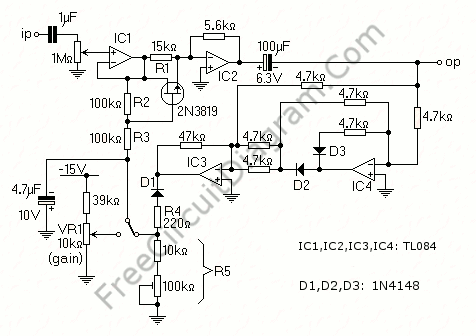## TC9400 Analog Division (Ratiometric Measurement)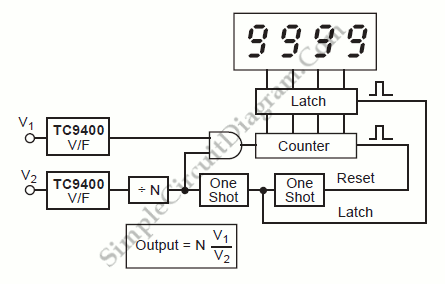A circuit which will divide one analog signal by another is one of the most difficult circuits to build.  TC9400 voltage-to-frequency converter make it easy to build such circuit.  Here is the block diagram of the system: Two voltage-to-frequency (V/F) converters can do such division with ease. While the denominator forms the time base, the numerator is counted directly as […]

## Twin-T Notch Filter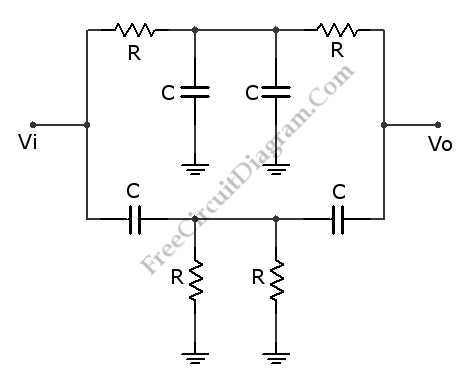This is a Twin-T Notch Filter circuit. This circuit is a filter that can remove a certain frequency band. This is a passive filter that uses two filters in parallel, high-pass and low-pass. The high-pass filter is lower one and the low-pass filter is the upper one. This filter has a problem with the input resistance. When this filter is […]

## Complement Phase Inverter with Single Transistor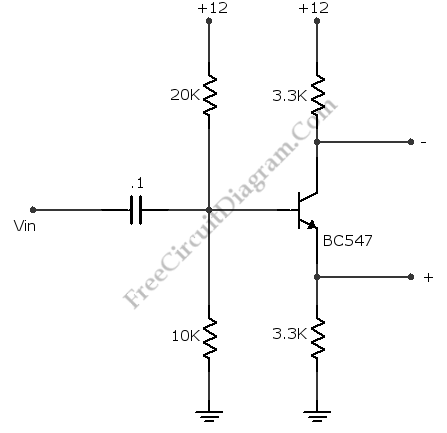This is a complement phase inverter circuit. Sometimes this circuit called a paraphase amplifier circuit. This circuit has two output signals that is have sufficient “headroom” because this circuit is biased. The transistor will be saturated when the collector decreases, the emitter voltage increases and set the limit. Here is the circuit: This circuit can produces a peak to peak […]

## Differentiator Operator with Op-Amp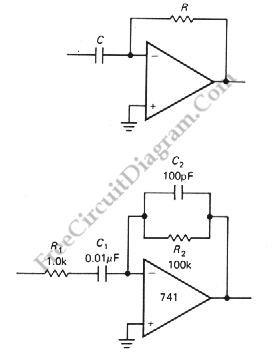Another application of OP-AMP is differentiator. Differentiator circuit produce an output if the input is changing. The output can positive or negative, depends on the change of the input: whether it’s decreasing or increasing.  This circuit is a bias stable. The current is produced by the rate of change of input voltage because the inverting input is grounded. Here is […]

## Summing Amplifier with Op-Amp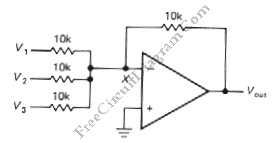One of inverting amplifier application is for summing several electric signals. The output of this circuit produce the sums of the input voltages.  We can use both positive or negative voltage for the inputs. The input resistors doesn’t have to be  same values. The input can be expanded to several digits. Since the point X is a virtual ground, the […]

## Analog Tremolo (Guitar) Sound Effect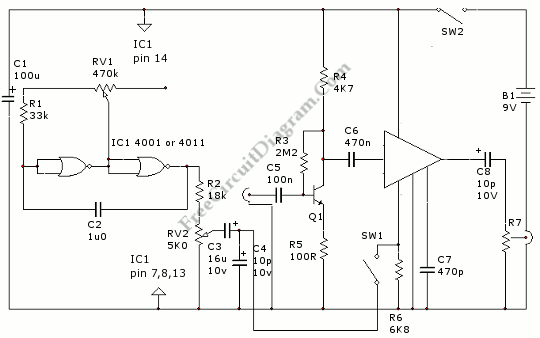Tremolo effect produce an amplitude modulated signal. Unlike vibrato effect that produce frequency modulated, this tremolo effect will produce the same effect as quickly turning up-ad-down your volume control repeatedly. Here is the schematic diagram of the circuit: Q1 is general small signal audio transistor, you can use almost any type of NPN small signal transistors. MFC3304P is a voltage […]